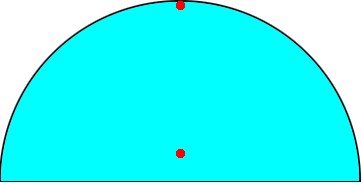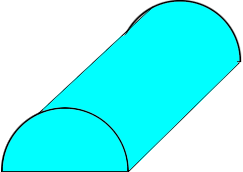SEARCH HOMEMath Central Quandaries & QueriesQuestion from Rohit, a student: why isn't volume of a sphere = Area of a semicircle x the circumference. i.e. if we revolve a semicircle around its axis we get a sphereHi Rohit,

Look at the semicircle below and imagine that it revolves around the horizontal axis to form a sphere.The distance travelled by the red dot at the top is almost the length of the circumference of the circumference of the sphere but the distance travelled by the red dot close to the center is much less.

If each point in the semicircle travels a distance which is the circumference then you obtain half a circular cylinderand its volume is area of the semicircle times the circumference.

PennyMath Central is supported by the University of Regina and The Pacific Institute for the Mathematical Sciences.